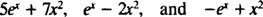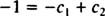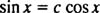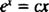## Linear Combinations, Linear Independence

Second‐order differential equations involve the second derivative of the unknown function (and, quite possibly, the first derivative as well) but no derivatives of higher order. For nearly every second‐order equation encountered in practice, the general solution will contain two arbitrary constants, so a second‐order IVP must include two initial conditions.

Given two functions y 1( x) and y 2( x), any expression of the formwhere c 1 and c 2 are constants, is called a linear combination of y 1 and y 2. For example, if y 1 = e x and y 2 = x 2, thenare all particular linear combinations of y 1 and y 2. So the idea of a linear combination of two functions is this: Multiply the functions by whatever constants you wish; then add the products.

Example 1: Is y = 2 x a linear combination of the functions y 1 = x and y 2 = x 2?

Any expression that can be written in the formis a linear combination of x and x 2. Since y = 2 x fits this form by taking c 1 = 2 and c 2 =o, y = 2 x is indeed a linear combination of x and x 2.

Example 2: Consider the three functions y 1 = sin x, y 2 = cos x, and y 3 = sin( x + 1). Show that y 3 is a linear combination of y 1 and y 2.

The addition formula for the since function saysNote that this fits the form of a linear combination of sin x and cos x,by taking c 1 = cos 1 and c 2 = sin 1.

Example 3: Can the function y = x 3 be written as a linear combination of the functions y 1 = x and y 2 = x 2?

If the answer were yes, then there would be constants c 1 and c 2 such that the equationholds true for all values of x. Letting x = 1 in this equation givesand letting x = −1 givesAdding these last two equations gives 0 = 2 c 2, so c 2 = 0. And since c 2 = 0, c 1 must equal 1. Thus, the general linear combination (*) reduces towhich clearly does not hold for all values of x. Therefore, it is not possible to write y = x 3 as a linear combination of y 1 = x and y 2 = x 2.

One more definition: Two functions y 1 and y 2 are said to be linearly independent if neither function is a constant multiple of the other. For example, the functions y 1 = x 3 and y 2 = 5 x 3 are not linearly independent (they're linearly dependent), since y 2 is clearly a constant multiple of y 1. Checking that two functions are dependent is easy; checking that they're independent takes a little more work.

Example 4: Are the functions y 1( x) = sin x and y 2( x) = cos x linearly independent?

If they weren't, then y 1 would be a constant multiple of y 2; that is, the equationwould hold for some constant c and for all x. But substituting x = π/2, for example, yields the absurd statement 1 = 0. Therefore, the above equation cannot be true: y 1 = sin x is not a constant multiple of y 2 = cos x; thus, these functions are indeed linearly independent.

Example 5: Are the functions y 1 = e x and y 2 = x linearly independent?

If they weren't, then y 1 would be a constant multiple of y 2; that is, the equationwould hold for some constant c and for all x. But this cannot happen, since substituting x = 0, for example, yields the absurd statement 1 = 0. Therefore, y 1 = e x is not a constant multiple of y 2 = x; these two functions are linearly independent.

Example 6: Are the functions y 1 = xe x and y 2 = e x linearly independent?

A hasty conclusion might be to say no because y 1 is a multiple of y 2. But y 1 is not a constant multiple of y 2, so these functions truly are independent. (You may find it instructive to prove they're independent by the same kind of argument used in the previous two examples.)

Back to Top
A18ACD436D5A3997E3DA2573E3FD792A